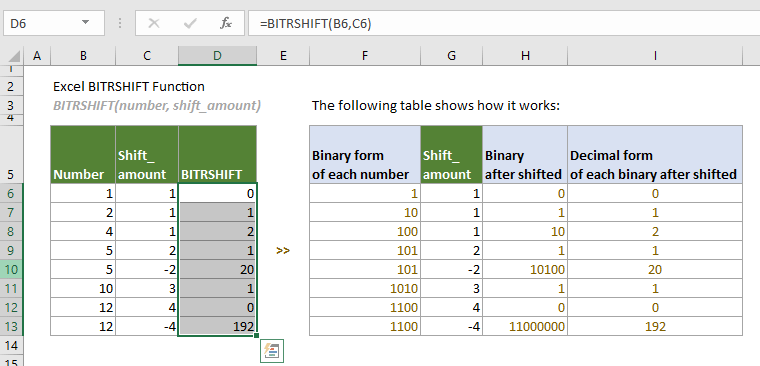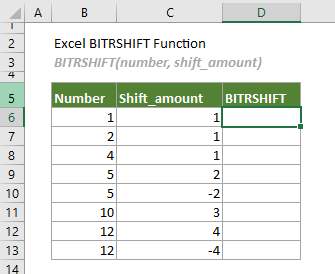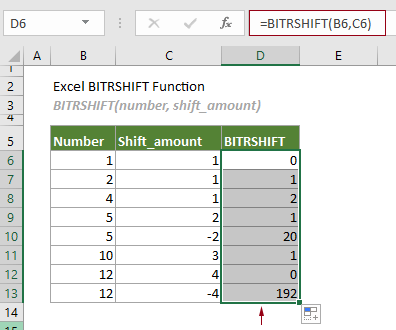## Excel BITRSHIFT function

The BITRSHIFT function returns the given number shifted right by the specified number of bits.

Note: This function is only available in Excel 2013 and later versions.#### Syntax

BITRSHIFT(number, shift_amount)

#### Arguments

• Number (required): This argument must be in decimal form greater than or equal to 0;
• Shift_amount (required): An integer represents the number of bits to be shifted. It allows positive integer and negative integer:
-- Positive integer: it shifts the number of bits to the right.
-- Negative integer: it shifts the number of bits to the left.

#### Remarks

1. The BITRSHIFT function shifts bits to the right when Shift_amount is supplied as a positive integer, which means that the specified number of digits will be removed from the rightmost side of the binary.
-- For example, shifting a decimal value of 12 by 4 bits, since the binary form of 12 is 1100, after shifting 4 bits to the right (like removing 4 digits from the rightmost side of the binary), the finally binary value is 0, which is also 0 in decimal.
2. The BITRSHIFT function shifts bits to the left when Shift_amount is supplied as a negative integer, which means that the specified number of digits will be added to the right of the binary.
-- For example, shifting a decimal value of 12 to the left by 4 bits, since the binary form of 12 is 1100, after shifting 4 bits to the left (like adding 4 digits to the right of the binary), the finally binary value is 1100000, which is 192 in decimal.
3. Arguments can be either of:
-- Numbers;
-- Cell references that contain numbers.
4. The #NUM! error value occurs when either of the following conditions is met:
-- Any of the supplied numbers is not an integer;
-- Any of the supplied numbers is greater than (2^48)-1.
5. The #VALUE! error value occurs if either of the supplied numbers is non-numeric.

#### Return value

It returns a numeric value.

#### Example

To shift the decimal numbers in column B by the number of bits listed in column C as shown in the table below, you can do as follows.Select a cell (says D6 in this case), enter the formula below and press Enter to get the first result. Select this result cell and then drag its AutoFill Handle down to get other results.

=BITRSHIFT(B6,C6)Notes:

1) Arguments in the formula above are supplied as cell references that contain numbers. However, you can change the formula in D6 as follows:

=BITRSHIFT(1,1)

2) How does this BITRSHIFT function work?

Take the formula in D6 as an example: =BITRSHIFT(B6,C6)

2.1) First, the function converts the decimal number to binary. .
In this case, the binary form of the decimal number ‘1’ is 1.
Tips: You can apply the DEC2BIN function to easily convert a decimal number to binary in Excel.
2.2) Then the binary was shifted right by the specified number of bits.
In this case, the binary 1 was shifted right by 1-bit the 0 was returned as the result.
2.3) Finally the function converts the binary number back to a decimal form.
In this case, the binary number 0 is returned in decimal form as an integer number 0.
Tips: You can apply the BIN2DEC function to easily convert a binary to decimal in Excel.

The following table shows how this function works in details.#### Related Functions

Excel BITOR function
The BITOR function returns the bitwise ‘OR’ of two given numbers.

Excel BITXOR function
The BITXOR function returns the bitwise ‘XOR’ of two given numbers.

Excel BITLSHIFT Function
The BITLSHIFT function returns a decimal number shifted left by a specified number of bits.

Excel BITAND function
The BITAND function returns a decimal number representing the bitwise 'AND' of two supplied numbers.

### The Best Office Productivity Tools

#### Kutools for Excel - Helps You To Stand Out From Crowd

 Popular Features: Find, Highlight or Identify Duplicates  |  Delete Blank Rows  |  Combine Columns or Cells without Losing Data  |  Round without Formula ... Super VLookup: Multiple Criteria  |  Multiple Value  |  Across Multi-Sheets  |  Fuzzy Lookup... Adv. Drop-down List: Easy Drop Down List  |  Dependent Drop Down List  |  Multi-select Drop Down List... Column Manager: Add a Specific Number of Columns  |  Move Columns  |  Toggle Visibility Status of Hidden Columns  |  Compare Columns to Select Same & Different Cells ... Featured Features: Grid Focus  |  Design View  |  Big Formula Bar  |  Workbook & Sheet Manager | Resource Library (Auto Text)  |  Date Picker  |  Combine Worksheets  |  Encrypt/Decrypt Cells  |  Send Emails by List  |  Super Filter  |  Special Filter (filter bold/italic/strikethrough...) ... Top 15 Toolsets:  12 Text Tools (Add Text, Remove Characters ...)  |  50+ Chart Types (Gantt Chart ...)  |  40+ Practical Formulas (Calculate age based on birthday ...)  |  19 Insertion Tools (Insert QR Code, Insert Picture from Path ...)  |  12 Conversion Tools (Numbers to Words, Currency Conversion ...)  |  7 Merge & Split Tools (Advanced Combine Rows, Split Excel Cells ...)  |  ... and more

Kutools for Excel Boasts Over 300 Features, Ensuring That What You Need is Just A Click Away...#### Office Tab - Enable Tabbed Reading and Editing in Microsoft Office (include Excel)

• One second to switch between dozens of open documents!
• Reduce hundreds of mouse clicks for you every day, say goodbye to mouse hand.
• Increases your productivity by 50% when viewing and editing multiple documents.
• Brings Efficient Tabs to Office (include Excel), Just Like Chrome, Edge and Firefox.